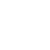Classes (extension) | UGens

# GaussClass : UGen : AbstractFunction : ObjectExtension

Gaussian classifier

## Description

A Gaussian classifier, which classifies an input vector as belonging to one of the gaussian distributions defined in a specially-formatted Buffer.

The Buffer should be single-channel. Its exact format is specified towards the bottom of this file. If you have the MathLib quark installed then you can use the convenience function GaussClass.classesToFloatArray() to create a FloatArray suitable for loading to a Buffer.

## Class Methods

### GaussClass.kr(in, bufnum: 0, gate: 0)

#### Arguments:

 in input signal, a multichannel signal specifying a co-ordinate in the space (i.e. a vector). bufnum the buffer in which the shapes and weights of the gaussian components are specified. gate the classifier is only active when this is greater than 0 (otherwise, previous value is held constant). Its default value is 1.

## Examples

The following example creates two-dimensional data with three classes:

________________________________

THE FORMAT OF THE BUFFER:

The Buffer should be single-channel and hold data in the following order, once for each class:

- N floats: the mean vector;

- N*N floats: the inverse of the covariance matrix; and

- 1 float: the weight of the component divided by the square root of the determinant of the covariance matrix.

N is the dimensionality of the data space. The length of the Buffer is therefore C * (N*N + N + 1). GaussClass.kr will determine the number of classes from the size of the Buffer.